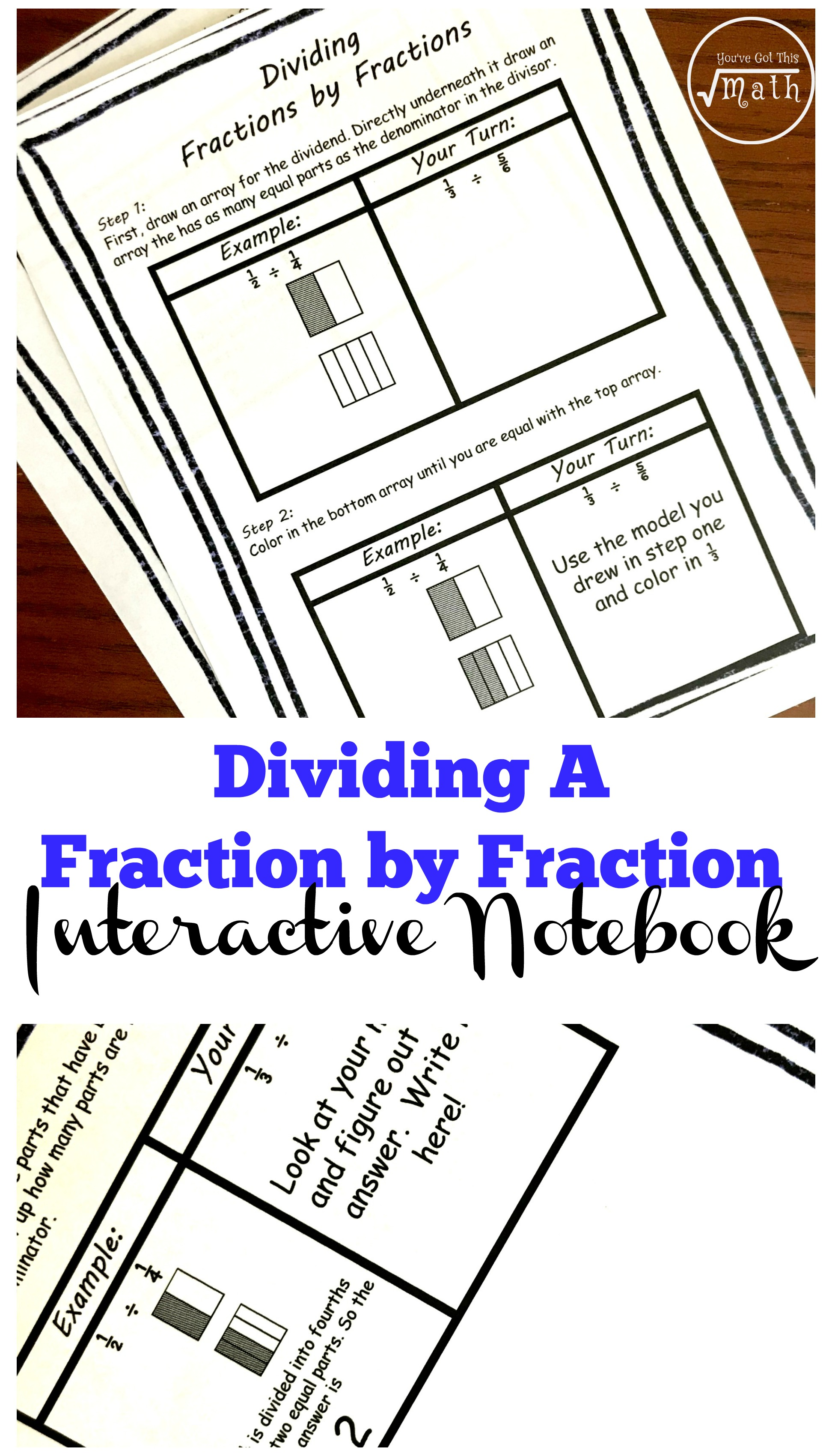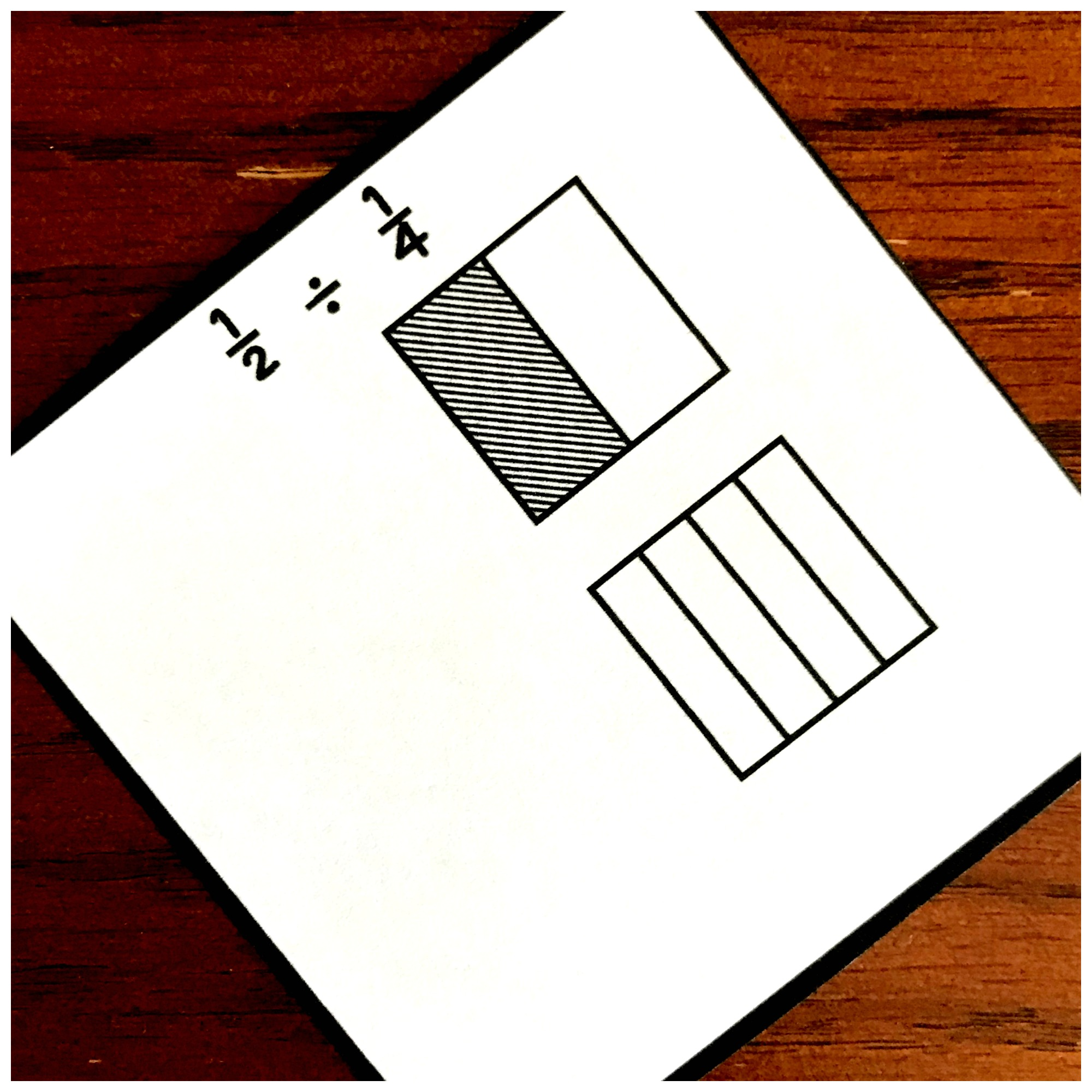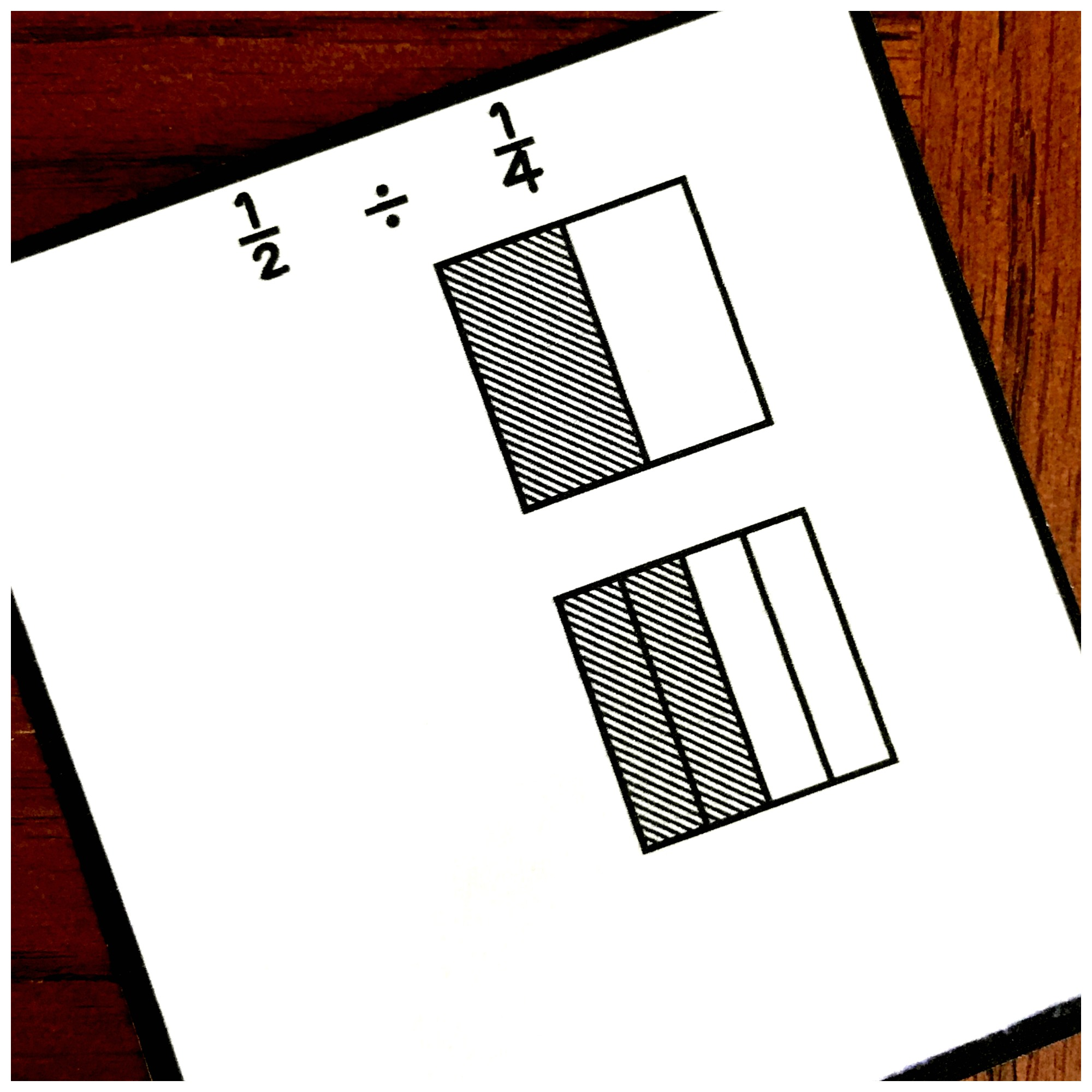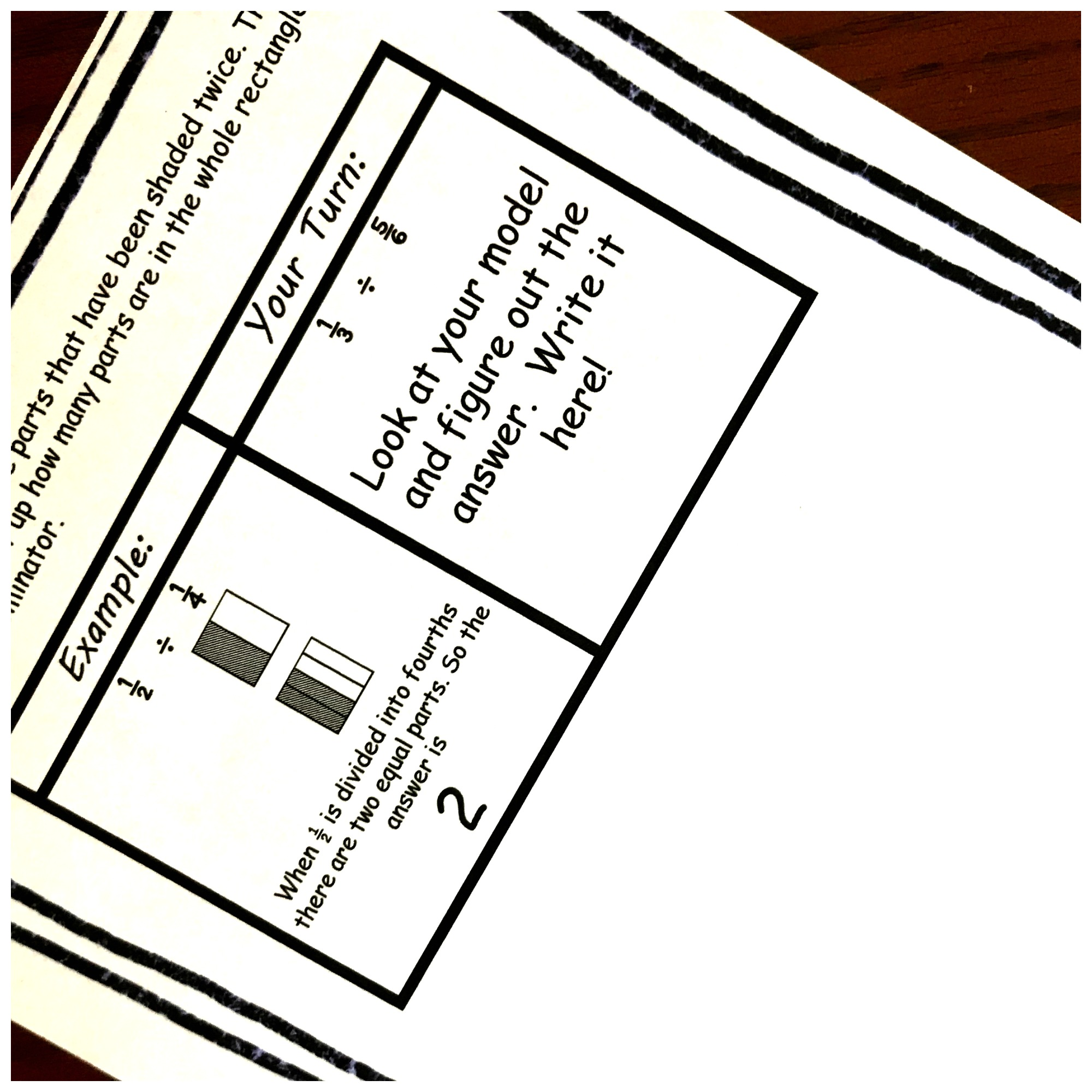Home » Math » FREE Interactive Notebook To Provide Dividing Fractions Examples

FREE Interactive Notebook To Provide Dividing Fractions Examples

This free interactive notebook provides dividing fractions examples using area models. An example is provided and then they have space for them to try it out!I’ll admit it has been awhile since I taught this one, and I had to do a little research.

Yes, I remember the whole reciprocal thing! But you know me. I want to always show my children the math they are doing….and so here we are.

This interactive notebook will provide your kiddos with dividing fractions examples and give them a chance to practice. The best part is that as they finish they will see what happens when you divide two fractions, and not just be able to do the operation.

*Before you begin, you may want to review division vocabulary! Your kiddos will need to know what a dividend is and what a divisor is!!

Step 1:First, draw an array for the dividend. Directly underneath it draw an array the has as many equal parts as the denominator in the divisor.

In this example, my dividend (the number being divided up) is one-half. You can see that I drew a model that equals one half. If I look at my divisor, it is one-fourth. So I’m going to take my next model and divide it up into fourths.

Step 2:Color in the bottom array until you are equal to the top array.

As you can see, I had to color in two-fourths so that the bottom model equals one-half.

Step 3:Finally, we count up how many parts we colored in. And that is our answer!

In this example, there are two fourths in one-half. We colored in two parts so that both models have the same amount shaded in. Therefore, the answer is 2.

In other words, if I divide one-half up into fourths, there will be two parts!

Prep- Work

This is a fun one that has very little prep -work.

1. First, you print off the interactive notebook for dividing fractions example
2. Next, provide glue, pencils, and colored pencils.
3. Finally, have your students glue the interactive notebook pages into a math notebook.Mead Spiral Notebook, 1 Subject, Quad Ruled Paper, 100 Sheets, 10-1/2Directions For Dividing Fractions Example Interactive NotebookWhat we walked through at the beginning of this post, is exactly what the interactive notebook walks your children through. Verbally, walk them through each step, and then let them try it.

When you are done, allow them more opportunities to practice this skill in their journal. Grabbing some fractions word problems that ask the children to model is a great place to start!! (I will be making some of those soon)

Or check out some of the dividing fractions resources on my 100 fractions activities project.